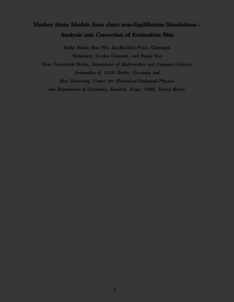Repository: Freie Universität Berlin, Math Department

# Markov State Models from short non-Equilibrium Simulations - Analysis and Correction of Estimation Bias

Nüske, F. and Wu, H. and Wehmeyer, C. and Clementi, C. and Noé, F. (2017) Markov State Models from short non-Equilibrium Simulations - Analysis and Correction of Estimation Bias. J. Chem. Phys., 146 . 094104.Preview

778kB

Official URL: http://dx.doi.org/10.1063/1.4976518

## Abstract

Many state-of-the-art methods for the thermodynamic and kinetic characterization of large and complex biomolecular systems by simulation rely on ensemble approaches, where data from large numbers of relatively short trajectories are integrated. In this context, Markov state models (MSMs) are extremely popular because they can be used to compute stationary quantities and long-time kinetics from ensembles of short simulations, provided that these short simulations are in “local equilibrium” within the MSM states. However, over the last 15 years since the inception of MSMs, it has been controversially discussed and not yet been answered how deviations from local equilibrium can be detected, whether these deviations induce a practical bias in MSM estimation, and how to correct for them. In this paper, we address these issues: We systematically analyze the estimation of MSMs from short non-equilibrium simulations, and we provide an expression for the error between unbiased transition probabilities and the expected estimate from many short simulations. We show that the unbiased MSM estimate can be obtained even from relatively short non-equilibrium simulations in the limit of long lag times and good discretization. Further, we exploit observable operator model (OOM) theory to derive an unbiased estimator for the MSM transition matrix that corrects for the effect of starting out of equilibrium, even when short lag times are used. Finally, we show how the OOM framework can be used to estimate the exact eigenvalues or relaxation time scales of the system without estimating an MSM transition matrix, which allows us to practically assess the discretization quality of the MSM. Applications to model systems and molecular dynamics simulation data of alanine dipeptide are included for illustration. The improved MSM estimator is implemented in PyEMMA of version 2.3.

Item Type: Article SFB 1114 Preprint 01/2017 in arXiv:1701:01665 Physical Sciences > PhysicsPhysical Sciences > ChemistryMathematical and Computer Sciences > Artificial Intelligence > Machine Learning Department of Mathematics and Computer Science > Institute of Mathematics > Comp. Molecular Biology 2002 BioComp Admin 06 Jan 2017 18:09 30 Nov 2017 16:46

Repository Staff Only: item control page